# An algebraic study of the Klein Bottle

## Abstract

We use symbolic computation (SC) and homological perturbation (HPT) to compute a resolution of the integers $$\mathbb {Z}$$ over the integer group ring of G, the fundamental group of the Klein bottle. From this it is easy to read off the homology of the Klein bottle as well as other information.

## Introduction

This paper has been derived from an example given in a graduate course in Symbolic Computation (SC) given at Stockholm University in 1994. It is also an introduction to Homological Perturbation Theory (HPT) and homological algebra of the Klein Bottle. The material presented was originally a special case of a very general research project using SCRATCHPAD by the author when he worked at IBM, Yorktown Heights, NY with the SCRATCHPAD Group in the 1980s.

## Homological perturbation theory

A background reference for this subject is given in . Suggested references for chain complexes and homological algebra are  and . For chain complexes and homology, see .

### Strong deformation retracts

Let X and Y be chain complexes over a ring k,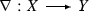,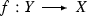be chain maps and let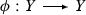be a degree one k-linear map such that $$f\nabla = 1_X$$ and $$d\phi +\phi d = 1 - \nabla f$$. Thus, f and $$\nabla$$ compose to the identity, but the composition the other way around is only chain homotopic to the identity. When these conditions hold, we say that this collection of data forms a strong deformation retraction (SDR) and we write

\begin{aligned} X Y \nabla f \phi . \end{aligned}
(1)

Important for certain computations are the side conditions 

\begin{aligned} \phi ^2 =0,\quad \phi \nabla =0,\quad \mathrm {and},\quad f\phi =0. \end{aligned}
(2)

In fact, these may always be assumed to hold: if the last two do not hold, replace $$\phi$$ by $$\phi ' = D(\phi )\phi D(\phi )$$ where $$D(\phi ) = \phi d + d\phi$$ and the last two conditions will now hold with respect to $$\phi '$$. If the first condition does not hold for $$\phi '$$, replace it by $$\phi '' = \phi ' d \phi '$$ and all three conditions will hold for the chain homotopy $$\phi ''$$. We will always assume that the side conditions hold when we talk about an SDR.

### The perturbation Lemma

Given the SDR (1) and, in addition, a second differential $$d_Y'$$ on Y, let $$t = d_Y'-d_Y$$. The perturbation lemma, [24, 8] states that if we set $$t_n = (t\phi )^{n-1}t, \ n \ge 1$$ and, for each n, define new maps on X,

\begin{aligned} \partial _n&= d + f (t_1 + t_2 + \cdots + t_{n-1}) \nabla \\ \nabla _n&= \nabla + \phi (t_1 + t_2 + \cdots + t_{n-1}) \nabla , \end{aligned}

and on Y:

\begin{aligned} f_n&= f + f (t_1 + t_2 + \cdots + t_{n-1}) \phi \\ \phi _n&= \phi + \phi (t_1 + t_2 + \cdots + t_{n-1}) \phi , \end{aligned}

then in the limits, provided they exist, we have new SDR data

Note that the limits will certainly exist if $$t\phi$$ is nilpotent in each degree. Examples relevant to this paper may be found in .

### Remark 2.1

In setting up a situation as above, it is useful to think of the perturbation as transferring the new differential $$d'$$ on a larger complex to a smaller one. In this sense, the data is said to be a transference problem and t is called the initiator. This notation is from  and its use is continued here.

## Resolutions

For a module N over an algebra A with ground ring k, we will generally consider resolutions of N over A of the form $$X = A \otimes \overline{X}$$ where $$\overline{X}$$ is free over k [5, 6]. Such complexes are called relatively free . Furthermore, it will be assumed that there is an explicit SDR where $$\epsilon$$ is an A-linear map, but generally, $$\sigma$$ and $$\psi$$ are only k-linear.

## FriCAS

SCRATCHPAD was a research project at IBM, Yorktown Hts, NY which was bought and productized by the Numeric Algorithms group in the early 1990s. At that time, it was renamed AXIOM. An introduction, well suited to research mathematicians is given in  and  while a general reference is given in . The system has subsequently become an open source project and several branches have formed. The one this author works with is called FriCAS .

The main idea to take away from this paper is that one can represent the class function part of a functor from category theory as a “parameterized type” or “constructor”. For example, if a monoid M and a ring R have been implemented, then there is a constructor MonoidRing(RM) giving the monoid ring of M over R. With this kind of construct, it is convenient to implement, for example, the bar construction of a monoid over an augmented ring using the data structure

\begin{aligned} Bar(R,M) = FreeModule (MonoidRing (R,M), List (M)). \end{aligned}

So for example, one can get the integer bar construction of the free group on n elements over the integers as $$bar = Bar(Z, FreeAbelian (n))$$. This constructor and several others are used in our calculations below.

## The Klein bottle

The Klein bottle may be described using identifications of the sides of a rectangle as shown in Fig. 1.

The vertical identifications give a cylinder and if the horizontal identifications were in the same direction, one would have a torus, but since they are reversed, one has a sort of “twisted torus”. Such an object cannot be embedded in three-space, but requires at least four dimensions. However, Fig. 2 gives an emersion of the bottle in three-space.Footnote 1

As mentioned above, the Klein Bottle K is a kind of twisted torus. As such, it is a non-trivial circle fibration over a circle: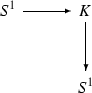(3)

Taking fundamental groups, one obtains a split exact sequence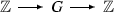(4)

for the fundamental group G of K. Since K is a K(G, 1) [19, 20], the homology of G is the homology of K, but we will go further than that in the next section.

## A resolution of $$\mathbf {\mathbb {Z}}$$ over the integer group ring of $$\mathbf {G}$$

In this section, a transference problem will be set up. From above, G is a non-trivial semi-direct product of $$\mathbb {Z}$$ with itself and the only non-trivial morphism from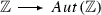is given by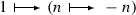, as a group, G is isomorphic to $$\mathbb {Z}^2$$ with the operation

\begin{aligned} x \cdot y = (x_1 + (-1)^{x_2} y_1, x_2 + y_2) = x+y + P(x,y) \end{aligned}
(5)

where $$x = (x_1,x_2), y = (y_1,y_2) \in {\mathbbm {Z^2}}$$ and P is a perturbation of the usual free abelian group law.

Let $$A = \mathbb {Z}(G)$$ and $$A_0 = \mathbb {Z}(\mathbb {Z}^2)$$. A well-known resolution of $$\mathbb {Z}$$ over $$A_0$$ is given by the Koszul–Tate resolution, where we consider $$\mathbb {Z}^2$$ as the free abelian group generated by elements $$t_1$$ and $$t_2$$: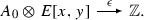(6)

(one extends the $$A_0$$-linear map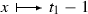and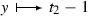as a graded derivation ). It can be shown that one has an SDR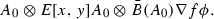(7)

where $$A_0 \otimes \bar{B}(A_0)$$ is the bar construction (see  for the bar construction). This is a special case of what is constructed in .

Now additively, i.e. as $$\mathbb {Z}$$ modules, $$B(A_0) = A_0 \otimes \bar{B}(A_0)$$ may be identified with $$B(G) = A \otimes \bar{B}(A)$$. Thus, we have a transference problem for the SDR (7) using the initiator $$t = \partial _G - \partial _{\mathbbm {Z^2}}$$ where $$\partial$$ denotes the differentials in the corresponding bar constructions (both considered as being on $$B(A_0)$$).

## The algorithm and computation

### The algorithm

Let z be an element in the Koszul-Tate resolution above and let b_ans_1, t_ans, ans t_phi, and d_inf be in the bar construction above. Also let t_phi = $$t \phi$$ and d denote the differential in the Tate-Koszul resolution. The following is pseudo-code for the perturbation lemma:

### The computation

The results of executing the algorithm above give the desired resolution. We have the A-linear differential

\begin{aligned} d(1)= & {} 0 \end{aligned}
(8)
\begin{aligned} d(x)= & {} t_1-1 \end{aligned}
(9)
\begin{aligned} d(y)= & {} t_2-1 \end{aligned}
(10)
\begin{aligned} d(xy)= & {} (-t_2-t_1^{-1})x + (-1+t_1^{-1})y. \end{aligned}
(11)

The reduced differential has only one non-zero term, viz.

\begin{aligned} d(u_1u_2) = -2u_1 \end{aligned}
(12)

which gives the correct homology for the Klein bottle

\begin{aligned} H_0(K)= & {} \mathbb {Z} \end{aligned}
(13)
\begin{aligned} H_1(K)= & {} \mathbb {Z} \oplus \mathbb {Z}/2 \end{aligned}
(14)
\begin{aligned} H_n(K)= & {} 0,\ n > 1. \end{aligned}
(15)

Note that one can also obtain the contracting homotopy as well as cycle representatives of the homology classes in the bar construction using this method. More details in general are in .

### Remark 7.1

The computation above obviously showed that the perturbation converged, but an analytic proof is possible, in this case, due to the fact that we have a closed formula for the homotopy ; however, such a computation “by hand” are essentially equivalent to the computation above except that the computer automated it and the program hid the details. This is applicable to a wider class of examples such as the solvable group examples given in . In general, one must examine other specific classes on a case by case basis.

### Remark 7.2

For alternate methods of computing resolutions and homology for certain classes of groups the interested reader should see  and .

1. 1.

The author is grateful to the referee for supplying this graphic for the paper.

## References

1. 1.

Lambe, Larry, Stasheff, J.: Applications of perturbation theory to iterated fibrations. Manuscripta Math. 58(3), 363–376 (1987)

2. 2.

Brown, R.: The twisted Eilenberg-Zilber theorem. In Simposio di Topologia (Messina, 1964), pp. 33–37. Edizioni Oderisi, Gubbio (1965)

3. 3.

Gugenheim, V.K.A.M.: On the chain-complex of a fibration. Ill. J. Math. 16, 398–414 (1972)

4. 4.

Barnes, Donald W., Lambe, Larry A.: A fixed point approach to homological perturbation theory. Proc. Am. Math. Soc. 112(3), 881–892 (1991)

5. 5.

Mac Lane, S.: Homology. Classics in Mathematics. Springer, Berlin. Reprint of the 1975 edition (1995)

6. 6.

Cartan, H., Eilenberg, S.: Homological Algebra. Princeton University Press, Princeton (1956)

7. 7.

Hilton, P.J., Wylie, S.: Homology theory: an introduction to algebraic topology. Cambridge University Press, New York (1960)

8. 8.

Lambe, L.A.: Entry on Homological Perturbation Theory in the Encyclopaedia of Mathematics. Supplement II. Kluwer Academic Press, Dordrecht (2000)

9. 9.

Lambe, Larry A.: Resolutions via homological perturbation. J. Symb. Comput. 12, 71–87 (1991)

10. 10.

Lambe, L.A.: Homological perturbation theory, Hochschild homology, and formal groups. In: Deformation theory and quantum groups with applications to mathematical physics (Amherst, MA, 1990), vol. 134 of Contemp. Math., pp. 183–218. Am. Math. Soc., Providence, RI (1992)

11. 11.

Lambe, Larry A.: Resolutions which split off of the bar construction. J. Pure Appl. Algebra 84(3), 311–329 (1993)

12. 12.

Lambe, L: Next generation computer algebra systems AXIOM and the scratchpad concept: applications to research in algebra. In: Analysis, algebra, and computers in mathematical research (Luleå, 1992), Lecture Notes in Pure and Appl. Math., vol. 156, pp. 201–222. Dekker, New York (1994)

13. 13.

Lambe, L.A., Grabmeier, J: Computing resolutions over finite $$p$$-groups. Algebraic combinatorics and applications. In: Betten, A., Kohnert, A., Laue, R., Wassermann, A. (eds.)Proceedings of the Euroconference (ALCOMA) held in Gweinstein, September 12–19 (1999)

14. 14.

Lambe, Larry A.: Scratchpad II as a tool for mathematical research. Not. Am. Math. Soc. 36, 141–148 (1989)

15. 15.

Lambe, Larry A.: The AXIOM system. Not. Am. Math. Soc. 41, 14–18 (1994)

16. 16.

Jenks, R.D., Sutor, R.S.: AXIOM Numerical Algorithms Group Ltd., Oxford (1992)

17. 17.

FriCAS. http://fricas.sourceforge.net/. Accessed 04 Nov 2016

18. 18.

Asymptote: The Vector Graphics Language. http://asymptote.sourceforge.net/. Accessed 04 Nov 2016

19. 19.

Eilenberg, S., MacLane, S.: Relations between homology and homotopy groups of spaces. Ann. Math. 46, 480–509 (1945)

20. 20.

Eilenberg, S., MacLane, S.: Relations between homology and homotopy groups of spaces II. Ann. Math. 51, 514–533 (1950)

21. 21.

Tate, J.: Homology of Noetherian rings and local rings. Ill. J. Math. 1, 14–27 (1957)

22. 22.

Ellis, G.: HAP–Homological Algebra Programming. http://www.gap-system.org/Packages/hap.html. Accessed 04 Nov 2016

23. 23.

Brady, T: Free resolutions for semi-direct products. Tohoku Math. J. (2) 45(4) 535–537 (1993)

## Author information

Authors

### Corresponding author

Correspondence to Larry A. Lambe.